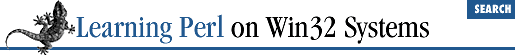home | O'Reilly's CD bookshelfs | FreeBSD | Linux | Cisco | Cisco Exam

### A.8 Chapter 9, Miscellaneous Control Structures

1. Here's one way to do it:

```sub card {} # from previous exercise

while () { ## NEW ##
print "Enter first number: ";
chomp(\$first = <STDIN>);

last if \$first eq "end"; ## NEW ##

print "Enter second number: ";
chomp(\$second = <STDIN>);
last if \$second eq "end"; ## NEW ##

\$message = &card(\$first) . " plus " .
&card(\$second) . " equals " .
&card(\$first+\$second) . ".\n";
print "\u\$message";
} ## NEW ##```

Note the addition of the ``` while``` loop, and the two ``` last``` operators. That's it!

2. Here's one way to do it:

```{
print "Enter a number (999 to quit): ";
chomp(\$n = <STDIN>);
last if \$n == 999;
\$sum += \$n;
redo;
}

print "the sum is \$sum\n";```

We're using a naked block with a ``` redo``` and a ``` last``` to get things done this time. We start by printing the prompt and grabbing the number. If it's 999, we exit the block with ``` last``` and print out the sum on exit. Otherwise, we add to our running total and use ``` redo``` to execute the block again.A.7 Chapter 8, FunctionsA.9 Chapter 10, Filehandles and File Tests